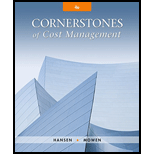# Refer to Cornerstone Exercise 7.7. Assume that Orchard Fresh, Inc., uses the weighted average method of joint cost allocation and has assigned the following weights to the four grades of apples: Total joint cost is $18,000. Required: 1. Allocate the joint cost to the four grades of apples using the weighted average method. (Carry out the percent calculations to four significant digits. Round all cost allocations to the nearest dollar.) 2. What if the factory found that Grade A apples were being valued less by customers and decided to decrease the weight factor for Grade A apples to 3.0? How would that affect the allocation of cost to Grade A apples? How would it affect the allocation of cost to the remaining grades?BuyFindarrow_forward ### Cornerstones of Cost Management (C... 4th Edition Don R. Hansen + 1 other Publisher: Cengage Learning ISBN: 9781305970663 #### Solutions Chapter SectionBuyFindarrow_forward ### Cornerstones of Cost Management (C... 4th Edition Don R. Hansen + 1 other Publisher: Cengage Learning ISBN: 9781305970663 Chapter 7, Problem 8CE Textbook Problem 60 views ## Refer to Cornerstone Exercise 7.7. Assume that Orchard Fresh, Inc., uses the weighted average method of joint cost allocation and has assigned the following weights to the four grades of apples:Total joint cost is$18,000.Required: 1. Allocate the joint cost to the four grades of apples using the weighted average method. (Carry out the percent calculations to four significant digits. Round all cost allocations to the nearest dollar.) 2. What if the factory found that Grade A apples were being valued less by customers and decided to decrease the weight factor for Grade A apples to 3.0? How would that affect the allocation of cost to Grade A apples? How would it affect the allocation of cost to the remaining grades?

1.

To determine

Allocate the joint costs to the four grades of apples using the weighted average method.

### Explanation of Solution

Joint cost: The total cost incurred to produce or manufacture or process, more than two products at the same time, is referred to as joint cost.

Allocate the joint costs:

 Grades Number of Pounds (a) Weight Factor (b) Weighted Number of Pounds (c = a × b) Percent (d) Allocated Joint Cost [(d) × $18,000] Grade A 1,600 4 6,400 (1)0.2362$4,252 Grade B 5,000 2 10,000 (1)0.369 \$6,642 Slices 8,000 1 8,000 (1)0

2.

To determine

Explain the situation if the factory found that Grade A apples are being valued less by customers and decided to decrease the weight factor for Grade A apples to 3.0, explain the manner in which it affects the allocation of cost to Grade A apples and explain the manner in which it affects the allocation of cost to the remaining grades.

### Still sussing out bartleby?

Check out a sample textbook solution.

See a sample solution

#### The Solution to Your Study Problems

Bartleby provides explanations to thousands of textbook problems written by our experts, many with advanced degrees!

Get Started